18

# Bowtie算法第六讲-tally法对bwt索引进行搜索

perl rotation_one_by_one.pl atgtgtcgtagctcgtnncgt

\$ATGTGTCGTAGCTCGTNNCGT 21

AGCTCGTNNCGT\$ATGTGTCGT 9

ATGTGTCGTAGCTCGTNNCGT\$ 0

CGT\$ATGTGTCGTAGCTCGTNN 18

CGTAGCTCGTNNCGT\$ATGTGT 6

CGTNNCGT\$ATGTGTCGTAGCT 13

CTCGTNNCGT\$ATGTGTCGTAG 11

GCTCGTNNCGT\$ATGTGTCGTA 10

GT\$ATGTGTCGTAGCTCGTNNC 19

GTAGCTCGTNNCGT\$ATGTGTC 7

GTCGTAGCTCGTNNCGT\$ATGT 4

GTGTCGTAGCTCGTNNCGT\$AT 2

GTNNCGT\$ATGTGTCGTAGCTC 14

NCGT\$ATGTGTCGTAGCTCGTN 17

NNCGT\$ATGTGTCGTAGCTCGT 16

T\$ATGTGTCGTAGCTCGTNNCG 20

TAGCTCGTNNCGT\$ATGTGTCG 8

TCGTAGCTCGTNNCGT\$ATGTG 5

TCGTNNCGT\$ATGTGTCGTAGC 12

TGTCGTAGCTCGTNNCGT\$ATG 3

TGTGTCGTAGCTCGTNNCGT\$A 1

TNNCGT\$ATGTGTCGTAGCTCG 15

T 21

T 9

\$ 0

N 18

T 6

T 13

G 11

A 10

C 19

C 7

T 4

T 2

C 14

N 17

T 16

G 20

G 8

G 5

C 12

G 3

A 1

G 15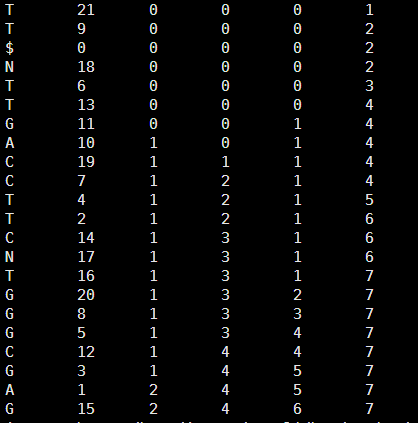perl search_char.pl    GTGTCG   tm.tally

your last char is G

start is 7 ; and end is 13

now it is number 5 and the char is C

start is 3 ; and end is 6

now it is number 4 and the char is T

start is 17 ; and end is 19

now it is number 3 and the char is G

start is 10 ; and end is 11

now it is number 2 and the char is T

start is 19 ; and end is 20

now it is number 1 and the char is G

start is 11 ; and end is 12

It is just one perfect match !

The index is 2

perl search_char.pl  GT  tm.tally

your last char is T

start is 15 ; and end is 22

now it is number 1 and the char is G

start is 8 ; and end is 13

we find more than one perfect match!!!

8 13

One of the index is 11

One of the index is 10

One of the index is 19

One of the index is 7

One of the index is 4

One of the index is 2

One of the index is 14

CTCGTNNCGT\$ATGTGTCGTAG 11

GCTCGTNNCGT\$ATGTGTCGTA 10

GT\$ATGTGTCGTAGCTCGTNNC 19

GTAGCTCGTNNCGT\$ATGTGTC 7

GTCGTAGCTCGTNNCGT\$ATGT 4

GTGTCGTAGCTCGTNNCGT\$AT 2

GTNNCGT\$ATGTGTCGTAGCTC 14

perl search_char.pl ACATGTGT tm.tally

your last char is T

start is 15 ; and end is 22

now it is number 7 and the char is G

start is 8 ; and end is 13

now it is number 6 and the char is T

start is 19 ; and end is 21

now it is number 5 and the char is G

start is 11 ; and end is 12

now it is number 4 and the char is T

start is 20 ; and end is 21

now it is number 3 and the char is A

start is 2 ; and end is 3

now it is number 2 and the char is C

start is 3 ; and end is 3

we can just find the last 6 char ,and it is ATGTGT

[perl]

\$a=\$ARGV;

\$a=uc \$a;

open FH,"<\$ARGV";

while(<FH>){

chomp;

@F=split;

\$hash_count_atcg{\$F}++;

\$hash{\$.}=\$_;

# the first line is \$ and the last char and the last index !

}

\$all_a=\$hash_count_atcg{'A'};

\$all_c=\$hash_count_atcg{'C'};

\$all_g=\$hash_count_atcg{'G'};

\$all_n=\$hash_count_atcg{'N'};

\$all_t=\$hash_count_atcg{'T'};

#print "\$all_a\t\$all_c\t\$all_g\t\$all_t\n";

\$len_a=length \$a;

\$end_a=\$len_a-1;

#print "your query is \$a\n";

#print "and the length of your query is \$len_a \n";

\$after=substr(\$a,\$end_a,1);

#we fill search your query from the last char !

if (\$after eq 'A') {

\$start=2;

\$end=\$all_a+1;

}

elsif (\$after eq 'C') {

\$start=\$all_a+1;

\$end=\$all_a+\$all_c+1;

}

elsif (\$after eq 'G') {

\$start=\$all_a+\$all_c+1;

\$end=\$all_a+\$all_c+\$all_g+1;

}

elsif (\$after eq 'T'){

\$start=\$all_a+\$all_c+\$all_g+\$all_n+1;

\$end=\$all_a+\$all_c+\$all_g+\$all_t+\$all_n+1;

}

else {die "error !!! we just need A T C G !!!\n"}

print "your last char is \$after\n ";

print "start is \$start ; and end is \$end \n";

foreach (reverse (1..\$end_a)){

\$after=substr(\$a,\$_,1);

\$before=substr(\$a,\$_-1,1);

(\$start,\$end)=&find_level(\$after,\$before,\$start,\$end);

print "now it is number \$_ and the char is \$before \n ";

print "start is \$start ; and end is \$end \n";

if (\$_  > 1 && \$start == \$end) {

\$find_char=substr(\$a,\$_);

\$find_len=length \$find_char;

print "we can just find the last \$find_len char ,and it is \$find_char \n";

#return "miss";

last;

}

if (\$_ == 1) {

if ((\$end-\$start)==1) {

print "It is just one perfect match ! \n";

my @F_start=split/\s+/,\$hash{\$end};

print "The index is \$F_start\n";

#return \$F_start;

last;

}

else {

print "we find more than one perfect match!!!\n";

print "\$start\t\$end\n";

foreach  ((\$start-1)..\$end) {

my @F_start=split/\s+/,\$hash{\$_};

print "One of the index is \$F_start\n";

}

#return "multiple";

last;

}

}

}

sub find_level{

my(\$after,\$before,\$start,\$end)=@_;

my @F_start=split/\s+/,\$hash{\$start};

my @F_end=split/\s+/,\$hash{\$end};

if (\$before eq 'A') {

return (\$F_start+1,\$F_end+1);

}

elsif (\$before eq 'C') {

return (\$all_a+\$F_start+1,\$all_a+\$F_end+1);

}

elsif (\$before eq 'G') {

return (\$all_a+\$all_c+1+\$F_start,\$all_a+\$all_c+1+\$F_end);

}

elsif (\$before eq 'T') {

return (\$all_a+\$all_c+\$all_g+\$all_n+1+\$F_start,\$all_a+\$all_c+\$all_g+1+\$all_n+\$F_end);

}

else {die "error !!! we just need A T C G !!!\n"}

}

[/perl]

18

# Bowtie算法第五讲-index2tally

\$ATGCGTANNGTC 12

ANNGTC\$ATGCGT 6

ATGCGTANNGTC\$ 0

C\$ATGCGTANNGT 11

CGTANNGTC\$ATG 3

GCGTANNGTC\$AT 2

GTANNGTC\$ATGC 4

GTC\$ATGCGTANN 9

NGTC\$ATGCGTAN 8

NNGTC\$ATGCGTA 7

TANNGTC\$ATGCG 5

TC\$ATGCGTANNG 10

TGCGTANNGTC\$A 1

C 12

T 6

\$ 0

T 11

G 3

T 2

C 4

N 9

N 8

A 7

G 5

G 10

A 1

[perl]

\$hash_count{'A'}=0;

\$hash_count{'C'}=0;

\$hash_count{'G'}=0;

\$hash_count{'T'}=0;

open FH ,"<\$ARGV";

while(<FH>){

chomp;

@F=split;

\$last=\$F; # 读取上面的tally文件，分列，判断第一列，并计数

\$hash_count{\$last}++;

print  "\$_\t\$hash_count{'A'}\t\$hash_count{'C'}\t\$hash_count{'G'}\t\$hash_count{'T'}\n";

}

[/perl]

C 12 0 1 0 0

T 6 0 1 0 1

\$ 0 0 1 0 1

T 11 0 1 0 2

G 3 0 1 1 2

T 2 0 1 1 3

C 4 0 2 1 3

N 9 0 2 1 3

N 8 0 2 1 3

A 7 1 2 1 3

G 5 1 2 2 3

G 10 1 2 3 3

A 1 2 2 3 3

18

# Bowtie 算法第四讲

[perl]

#首先读取大字符串的fasta文件

open FH ,"<\$ARGV";

\$i=0;

while (<FH>) {

next if /^>/;

chomp;

\$a.=(uc);

}

#print "\$a\n";

#然后接受我们的小的查询字符串

\$query=uc \$ARGV;

\$len=length \$a;

\$len_query=length \$query;

\$a=\$a.'\$'.\$a;

#然后依次循环取大字符串来精确比较！

foreach (0..\$len-1){

if (substr(\$a,\$_,\$len_query) eq \$query){

print "\$_\n";

#last;

}

}

[/perl]

[perl]

\$a=uc \$ARGV;

\$len=length \$a;

\$a=\$a.'\$'.\$a;

foreach (0..\$len){

\$hash{substr(\$a,\$_,\$len+1)}=\$_;

}

#print "\$_\t\$hash{\$_}\n" foreach sort keys %hash;

print  substr(\$_,-1),"\t\$hash{\$_}\n" foreach sort keys %hash;

[/perl]

perl rotation_one_by_one.pl atgcgtanngtc 这个字符串的BWT矩阵索引如下！

C 12

T 6

\$ 0

T 11

G 3

T 2

C 4

N 9

N 8

A 7

G 5

G 10

A 1

[perl]

#首先读取上面输出的BWT矩阵索引文件。

open FH,"<\$ARGV";

\$hash_count{'A'}=0;

\$hash_count{'C'}=0;

\$hash_count{'G'}=0;

\$hash_count{'T'}=0;

while(<FH>){

chomp;

@F=split;

\$hash_count{\$F}++;

\$hash{\$.}="\$F\t\$F\t\$hash_count{\$F}";

#print "\$hash{\$.}\n";

}

\$all_a=\$hash_count{'A'};

\$all_c=\$hash_count{'C'};

\$all_g=\$hash_count{'G'};

\$all_t=\$hash_count{'T'};

\$all_n=\$hash_count{'N'};

#start from the first char !

\$raw='';

&restore(1);

sub restore{

my(\$num)=@_;

my @F=split/\t/,\$hash{\$num};

\$raw.=\$F;

my \$before=\$F;

if (\$before eq 'A') {

\$new=\$F+1;

}

elsif (\$before eq 'C') {

\$new=1+\$all_a+\$F;

}

elsif (\$before eq 'G') {

\$new=1+\$all_a+\$all_c+\$F;

}

elsif (\$before eq 'N') {

\$new =1+\$all_a+\$all_c+\$all_g+\$F;

}

elsif (\$before eq 'T') {

\$new=1+\$all_a+\$all_c+\$all_g+\$all_n+\$F;

}

elsif (\$before eq '\$') {

chop \$raw;

\$raw = reverse \$raw;

print "\$raw\n";

exit;

}

else {die "error !!! we just need A T C N G !!!\n"}

#print "\$F\t\$new\n";

&restore(\$new);

}

[/perl]

28

# 自己动手写bowtie第三讲：序列查询。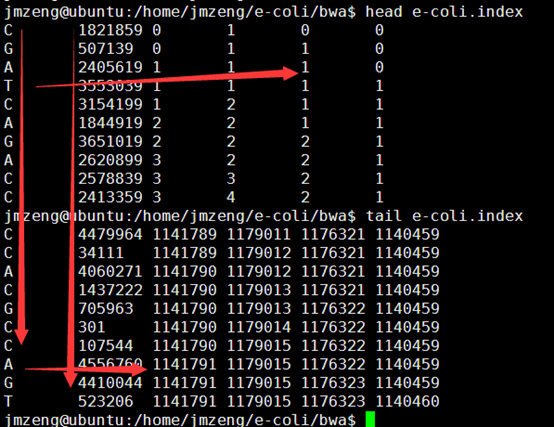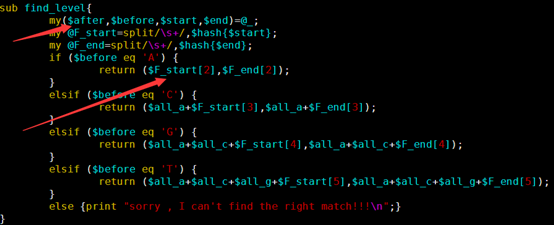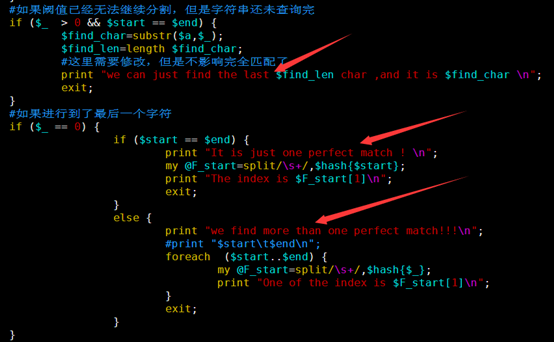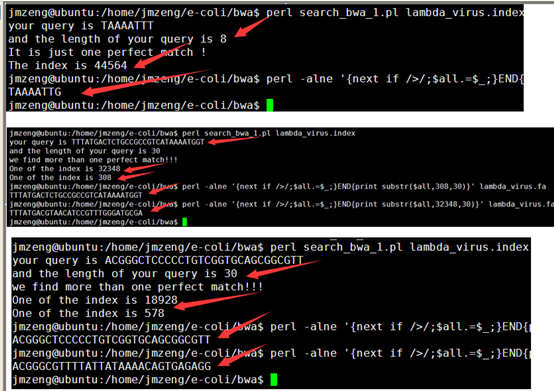[perl]

\$a='CGCTATGTACTGGATGCGCTGGCAAACGAGCCTGCCGTAAG';

while(<>){

chomp;

@F=split;

\$hash_count_atcg{\$F}++;

\$hash{\$.}=\$_;

}

\$all_a=\$hash_count_atcg{'A'};

\$all_c=\$hash_count_atcg{'C'};

\$all_g=\$hash_count_atcg{'G'};

\$all_t=\$hash_count_atcg{'T'};

#print "\$all_a\t\$all_c\t\$all_g\t\$all_t\n";

\$len_a=length \$a;

\$end_a=\$len_a-1;

print "your query is \$a\n";

print "and the length of your query is \$len_a \n";

foreach (reverse (0..\$end_a)){

\$after=substr(\$a,\$_,1);

\$before=substr(\$a,\$_-1,1);

#对第一个字符进行找阈值的时候，我们需要人为的定义起始点！

if(\$_ == \$end_a){

if (\$after eq 'A') {

\$start=1;

\$end=\$all_a;

}

elsif (\$after eq 'C') {

\$start=\$all_a+1;

\$end=\$all_a+\$all_c;

}

elsif (\$after eq 'G') {

\$start=\$all_a+\$all_c+1;

\$end=\$all_a+\$all_c+\$all_g;

}

elsif (\$after eq 'T'){

\$start=\$all_a+\$all_c+\$all_g+1;

\$end=\$all_a+\$all_c+\$all_g+\$all_t;

}

else {print "error !!! we just need A T C G !!!\n";exit;}

}

#如果阈值已经无法继续分割，但是字符串还未查询完

if (\$_  > 0 && \$start == \$end) {

\$find_char=substr(\$a,\$_);

\$find_len=length \$find_char;

#这里需要修改，但是不影响完全匹配了

print "we can just find the last \$find_len char ,and it is \$find_char \n";

exit;

}

#如果进行到了最后一个字符

if (\$_ == 0) {

if (\$start == \$end) {

print "It is just one perfect match ! \n";

my @F_start=split/\s+/,\$hash{\$start};

print "The index is \$F_start\n";

exit;

}

else {

print "we find more than one perfect match!!!\n";

#print "\$start\t\$end\n";

foreach  (\$start..\$end) {

my @F_start=split/\s+/,\$hash{\$_};

print "One of the index is \$F_start\n";

}

exit;

}

}

(\$start,\$end)=&find_level(\$after,\$before,\$start,\$end);

}

sub find_level{

my(\$after,\$before,\$start,\$end)=@_;

my @F_start=split/\s+/,\$hash{\$start};

my @F_end=split/\s+/,\$hash{\$end};

if (\$before eq 'A') {

return (\$F_start,\$F_end);

}

elsif (\$before eq 'C') {

return (\$all_a+\$F_start,\$all_a+\$F_end);

}

elsif (\$before eq 'G') {

return (\$all_a+\$all_c+\$F_start,\$all_a+\$all_c+\$F_end);

}

elsif (\$before eq 'T') {

return (\$all_a+\$all_c+\$all_g+\$F_start,\$all_a+\$all_c+\$all_g+\$F_end);

}

else {print "sorry , I can't find the right match!!!\n";}

}

#perl -alne '{next if />/;\$all.=\$_;}END{print substr(\$all,308,10)}'   lambda_virus.fa

[/perl]

28

# 自己动手写bowtie第二讲：优化索引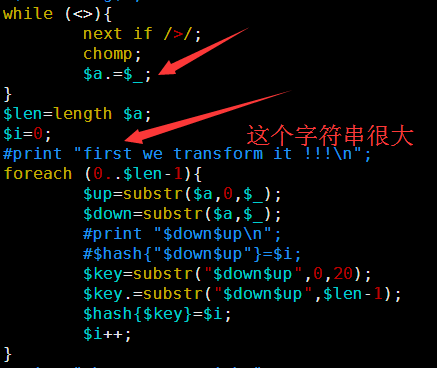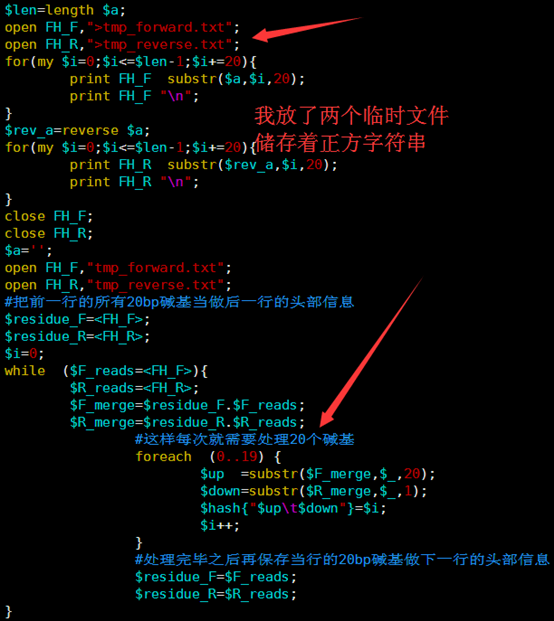time perl bwt_new_index.pl e-coli.fa >e-coli.index

real 0m43.071s

user 0m41.277s

sys 0m1.779s[perl]

while (<>){

next if />/;

chomp;

\$a.=\$_;

}

\$len=length \$a;

open FH_F,">tmp_forward.txt";

open FH_R,">tmp_reverse.txt";

for(my \$i=0;\$i<=\$len-1;\$i+=20){

print FH_F  substr(\$a,\$i,20);

print FH_F "\n";

}

\$rev_a=reverse \$a;

for(my \$i=0;\$i<=\$len-1;\$i+=20){

print FH_R  substr(\$rev_a,\$i,20);

print FH_R "\n";

}

close FH_F;

close FH_R;

\$a='';

open FH_F,"tmp_forward.txt";

open FH_R,"tmp_reverse.txt";

#把前一行的所有20bp碱基当做后一行的头部信息

\$residue_F=<FH_F>;

\$residue_R=<FH_R>;

\$i=0;

#这样每次就需要处理20个碱基

foreach  (0..19) {

\$up  =substr(\$F_merge,\$_,20);

\$down=substr(\$R_merge,\$_,1);

\$hash{"\$up\t\$down"}=\$i;

\$i++;

}

#处理完毕之后再保存当行的20bp碱基做下一行的头部信息

}

#print "then we sort it\n";

\$count_a=0;

\$count_c=0;

\$count_g=0;

\$count_t=0;

foreach  (sort keys  %hash){

\$first=substr(\$_,0,1);

\$len=length;

\$last=substr(\$_,\$len-1,1);

#print "\$first\t\$last\t\$hash{\$_}\n";

\$count_a++ if \$last eq 'A';

\$count_c++ if \$last eq 'C';

\$count_g++ if \$last eq 'G';

\$count_t++ if \$last eq 'T';

print "\$last\t\$hash{\$_}\t\$count_a\t\$count_c\t\$count_g\t\$count_t\n";

}